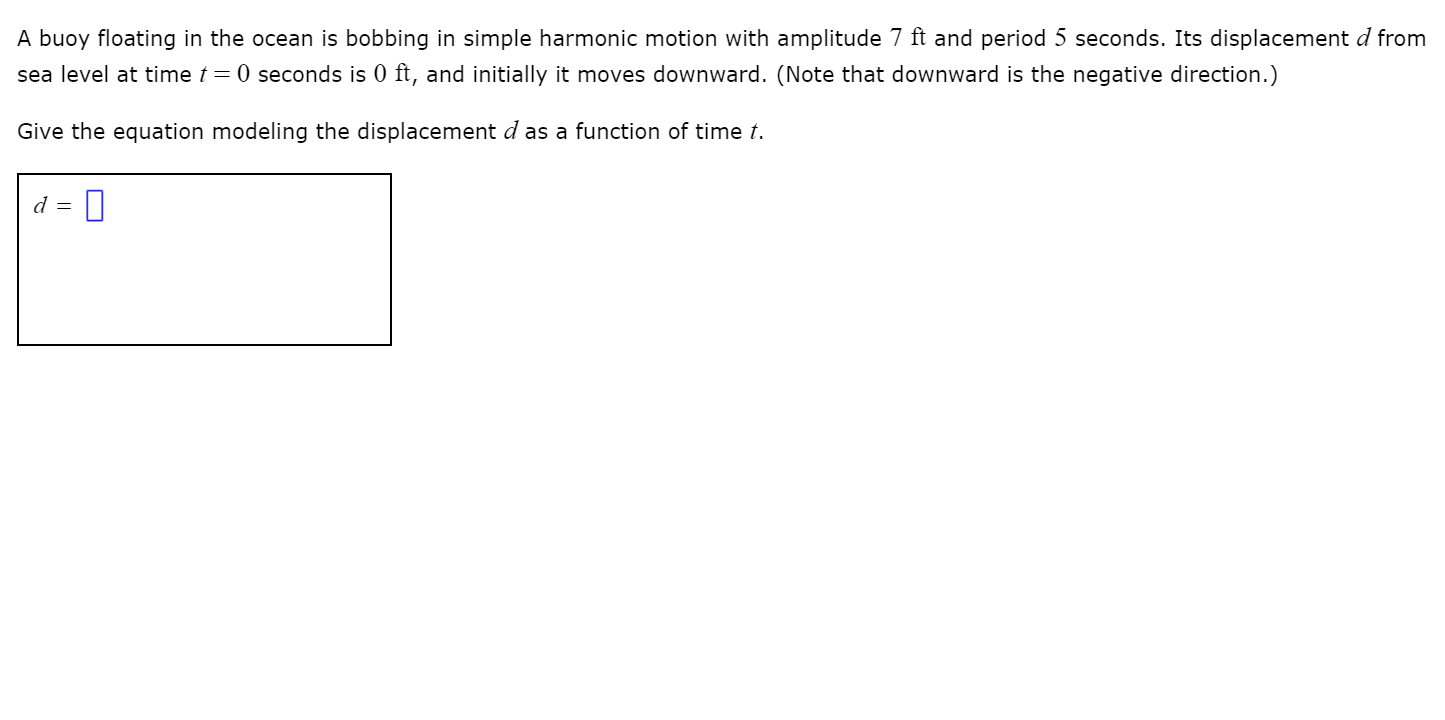# A buoy floating in the ocean is bobbing in simple harmonic motion with amplitude 7 ft and period 5 seconds. Its displacement d fromsea level at time t = 0 seconds is 0 ft, and initially it moves downward. (Note that downward is the negative direction.)Give the equation modeling the displacement d as a function of time t.

Question
297 viewshelp_outlineImage TranscriptioncloseA buoy floating in the ocean is bobbing in simple harmonic motion with amplitude 7 ft and period 5 seconds. Its displacement d from sea level at time t = 0 seconds is 0 ft, and initially it moves downward. (Note that downward is the negative direction.) Give the equation modeling the displacement d as a function of time t. fullscreen
check_circle

Step 1

Given,

Amplitude, a = 7 ft

Period = 5 seconds.

Since, d at t = 0 is 0 ft

Step 2

Now, at t = 0,...

### Want to see the full answer?

See Solution

#### Want to see this answer and more?

Solutions are written by subject experts who are available 24/7. Questions are typically answered within 1 hour.*

See Solution
*Response times may vary by subject and question.
Tagged in
MathCalculus

### Other